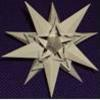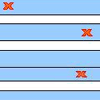Search by Topic

Resources tagged with Iteration similar to Climbing Powers:

Filter by: Content type:
Age range:
Challenge level:

There are 22 results

Broad Topics > Patterns, Sequences and Structure > IterationClimbing Powers

Age 16 to 18 Challenge Level:

$2\wedge 3\wedge 4$ could be $(2^3)^4$ or $2^{(3^4)}$. Does it make any difference? For both definitions, which is bigger: $r\wedge r\wedge r\wedge r\dots$ where the powers of $r$ go on for ever, or. . . .Archimedes Numerical Roots

Age 16 to 18 Challenge Level:

How did Archimedes calculate the lengths of the sides of the polygons which needed him to be able to calculate square roots?Difference Dynamics Discussion

Age 16 to 18

This article discusses what happens, and why, if you generate chains of sequences getting the next sequence from the differences between the adjacent terms in the sequence before it, eg (7, 2, 8, 3). . . .Route to Root

Age 16 to 18 Challenge Level:

A sequence of numbers x1, x2, x3, ... starts with x1 = 2, and, if you know any term xn, you can find the next term xn+1 using the formula: xn+1 = (xn + 3/xn)/2 . Calculate the first six terms of this. . . .Converging Means

Age 14 to 16 Challenge Level:

Take any two positive numbers. Calculate the arithmetic and geometric means. Repeat the calculations to generate a sequence of arithmetic means and geometric means. Make a note of what happens to the. . . .Triangle Incircle Iteration

Age 14 to 16 Challenge Level:

Keep constructing triangles in the incircle of the previous triangle. What happens?Loopy

Age 14 to 16 Challenge Level:

Investigate sequences given by $a_n = \frac{1+a_{n-1}}{a_{n-2}}$ for different choices of the first two terms. Make a conjecture about the behaviour of these sequences. Can you prove your conjecture?Stretching Fractions

Age 14 to 16 Challenge Level:

Imagine a strip with a mark somewhere along it. Fold it in the middle so that the bottom reaches back to the top. Stetch it out to match the original length. Now where's the mark?V-P Cycles

Age 16 to 18 Challenge Level:

Form a sequence of vectors by multiplying each vector (using vector products) by a constant vector to get the next one in the seuence(like a GP). What happens?Rain or Shine

Age 16 to 18 Challenge Level:

Predict future weather using the probability that tomorrow is wet given today is wet and the probability that tomorrow is wet given that today is dry.Infinite Continued Fractions

Age 16 to 18

In this article we are going to look at infinite continued fractions - continued fractions that do not terminate.Dalmatians

Age 14 to 18 Challenge Level:

Investigate the sequences obtained by starting with any positive 2 digit number (10a+b) and repeatedly using the rule 10a+b maps to 10b-a to get the next number in the sequence.Difference Dynamics

Age 14 to 18 Challenge Level:

Take three whole numbers. The differences between them give you three new numbers. Find the differences between the new numbers and keep repeating this. What happens?Ford Circles

Age 16 to 18 Challenge Level:

Can you find the link between these beautiful circle patterns and Farey Sequences?The Golden Ratio, Fibonacci Numbers and Continued Fractions.

Age 14 to 16

An iterative method for finding the value of the Golden Ratio with explanations of how this involves the ratios of Fibonacci numbers and continued fractions.Peaches in General

Age 14 to 16 Challenge Level:

It's like 'Peaches Today, Peaches Tomorrow' but interestingly generalized.Spirostars

Age 16 to 18 Challenge Level:

A spiropath is a sequence of connected line segments end to end taking different directions. The same spiropath is iterated. When does it cycle and when does it go on indefinitely?Slippage

Age 14 to 16 Challenge Level:

A ladder 3m long rests against a wall with one end a short distance from its base. Between the wall and the base of a ladder is a garden storage box 1m tall and 1m high. What is the maximum distance. . . .First Forward Into Logo 1: Square Five

Age 7 to 16 Challenge Level:

A Short introduction to using Logo. This is the first in a twelve part series.Stringing it Out

Age 14 to 16 Challenge Level:

Explore the transformations and comment on what you find.First Forward Into Logo 10: Count up - Count Down

Age 11 to 18 Challenge Level:

What happens when a procedure calls itself?Quorum-sensing

Age 16 to 18 Short Challenge Level:

This problem explores the biology behind Rudolph's glowing red nose.